Next: 7 Related Work Up: A Model for Decentralized Previous: 5 Application to a

# 6 Verification and Label Inference

This section describes code verification and label inference in more detail. It demonstrates that basic block labels, labels on local variables, and labels in `declassify` expressions can be inferred efficiently and automatically, making it much more convenient to write properly annotated code. We present a small example of this process.

## 6.1 Label Constraint Systems

As described earlier, procedure verification is a two-step process that first determines basic block labels by propagating the labels of branch-determining expressions, and then checking all the statements in the program in the context of their basic blocks. While the expressions that control branch decisions can be identified statically, their labels depend on the label of the basic block in which they occur. We seem to have a chicken-and-egg problem.

The difficulty can be resolved by creating a label constraint system and solving for all the inferred labels simultaneously. We start by inventing fresh labels Li for each of the branch expressions in blocks Bi. These labels are propagated according to the rules for determining basic block labels, and fed into the statement verification stage. Verifying each statement requires checking of corresponding constraints involving labels. To verify code, we collect all the constraints that are demanded by the various statements, and solve them as a system. If the constraints are inconsistent and generate a contradiction, the program contains an information leak.

Solving the constraint system is tractable because the constraints all take a particularly simple form. Each constraint has either a label or a join of labels on both the left- and right-hand sides, e.g., L1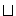L2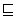L3L4. This equation is equivalent to the two equations L1L3L4 and L2L3L4, and in general, we can convert the constraints to a canonical form in which there is only one label (variable or constant) on the left-hand side.

Explicitly declared labels (whether constants or first-class labels) and implicit argument labels are treated as constants; basic block labels and undeclared labels on local variables are treated as variables. The goal of verification is to determine whether there are assignments to the local variable labels and basic block labels that satisfy all the constraints. This problem is similar in form to the problem of satisfying propositional Horn clauses; in fact, a linear-time algorithm for satisfying Horn clauses [DG84, RM96] can be adapted easily to this problem. If, on the other hand, we had permitted use of both the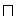andoperators in constructing label expressions, the label satisfaction problem would have become NP-complete [RM96].

The algorithm works by keeping track of conservative upper bounds for each unknown label. Initially, all the upper bounds are set to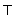. The algorithm then iteratively decreases the upper bounds, until either all equations are satisfied or a contradiction is observed. At each step, the algorithm picks an equation that is not satisfied when all variables are substituted by their upper bounds. If the unsatisfied equation has a constant label on its left-hand side, a contradiction has been detected. Otherwise, the upper bound estimate for the variable label on the left-hand side is adjusted to be the meet () of its current upper bound and the value of the right-hand side. In evaluating the right-hand side, all variables are replaced with their current upper bound estimates.

Like the algorithm for satisfying Horn clauses, this algorithm requires a number of iterations that is linear in the total size of the constraints; the total size of the constraints is at worst quadratic in the length of the code. Therefore, this inference algorithm seems very practical.

The labels found by this algorithm are the most restrictive labels that satisfy the constraints. However, the actual values that the inference algorithm finds are irrelevant, because they are never converted to first-class values of type `label`. What is important is that there is a satisfying assignment to all the labels, proving that the code is safe.

## 6.2 Inference Example

```check_password(db: array[pinfo{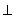}], user: string,
pwd: string)
returns (ret: bool{userpwddb})
% Return whether "pwd" is the password of "user"

i: int := 0				 %match: bool := false                 %while i < db.length() do             % L1
if db[i].names = user &            % L1
db[i].passwords = pwd then      %
match := true                 % L1L2
end                                %
i := i + 1                         % L1
end                                  %
ret := false                         %if_acts_for(check_password, chkr)    %
ret := declassify(match)           %end
end check_password
```
Figure 8: Password example with implicit labels

Figure 8 shows the code for a more flexible version of the `check_password` procedure that was presented earlier. This version of `check_password` is usable by any client principal. Because the arguments `db`, `user`, and `pwd` have no declared labels, their labels are implicit parameters to the routine. Note that the local variables `i` and `result` do not explicitly declare their labels. The resulting procedure is as safe as the previous version of `check_password`, and easier to implement and use. Let us now walk through the verification process for this code.

The first step in verification is to construct the basic-block diagram and propagate fresh labels that represent branch expressions. The comments in Figure 8 show the value of the basic block labels for each basic block, in terms of the two branch-expression labels L1 and L2 (for the `if` and `while`, respectively.)

Next, the code is analyzed to generate the set of label constraints shown in Figure 9, which include inequalities corresponding to the statements in the program, plus some equalities that bind the basic-block branch-expression labels to the labels for the corresponding expressions. The equalities can be transformed into a pair of constraints to preserve the canonical constraint form. Note that the use of `declassify` generates an additional constraint, introducing a new variable Ld that represents the label of the declassified result. This procedure provides a good example of why it is important for declassification to be able to apply to just one component of a join, as discussed in Section 3.4. The fact that declassification works at all in this procedure, let alone is possible to verify automatically, is due to this property of the declassification rule.

 `i := 0``i` `match := false``match` `while` `idb ` = L1 `if` ``` iuserpwddb{chkr: chkr}```L1```pwddb``` = L2 `match := true` L1L2`match` `i := i + 1` `i`L1`i` `ret := false````userpwddb ``` `declassify` `match`Ld`{chkr: Ø}` `ret := ...` Ld```userpwddb ```
Figure 9: Constraints for the password example

Applying the constraint-solving algorithm described above, a single backward pass through the canonical forms of these constraints yields labels that satisfy them, as shown in Figure 10.

 ```i, match, L1, L2 ``` = `user``pwd``db``{chkr: Ø}` Ld = `user``pwd``db`
Figure 10: Constraint solutions

Next: 7 Related Work Up: A Model for Decentralized Previous: 5 Application to a

Andrew C. Myers, Barbara Liskov